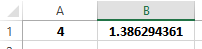### LN Function – Natural Logarithm – Excel, VBA & Google Sheets

This Tutorial demonstrates how to use the Excel LN Function in Excel to calculate the natural logarithm to a number.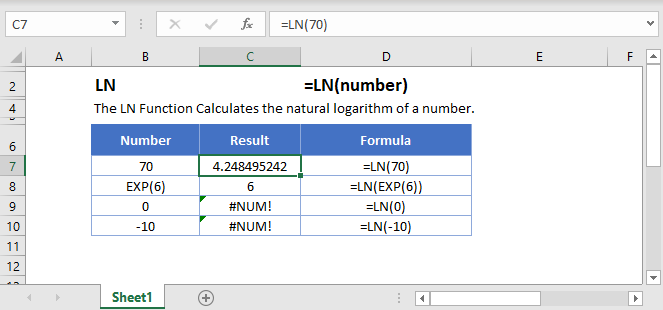## LN Function Overview

The LN Function Calculates the natural logarithm of a number.

To use the LN Excel Worksheet Function, select a cell and type: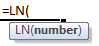(Notice how the formula inputs appear)

## LN Function Syntax and Inputs:

number – A number.

AutoMacro - VBA Code Generator

## LN Function

The LN Function returns the natural logarithm of a number entered.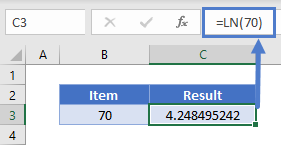## LN & EXP Function

The inverse of LN Function is EXP Function. If EXP Function is nested within LN Function, it will return the given argument as it is.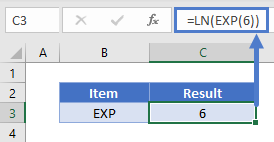Alternatively, the same observation can be made if the argument is given as a power of e.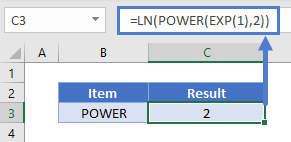Note: The number natural e cannot be directly entered in Excel so EXP(1) is used. You can explore the EXP Function here

## LN & LOG Function

The LN Function is the special case of LOG Function with the base e. LOG(4,e) will return the same result as LN(4).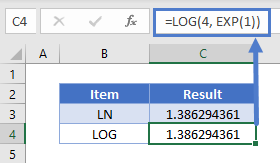## LN Function – Negative Number/Zero

The LN Function will return an error if the argument is zero or a negative number.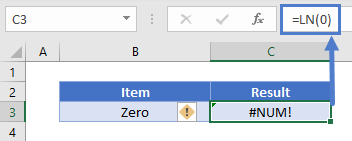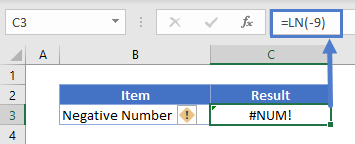The LN Function works exactly the same in Google Sheets as in Excel: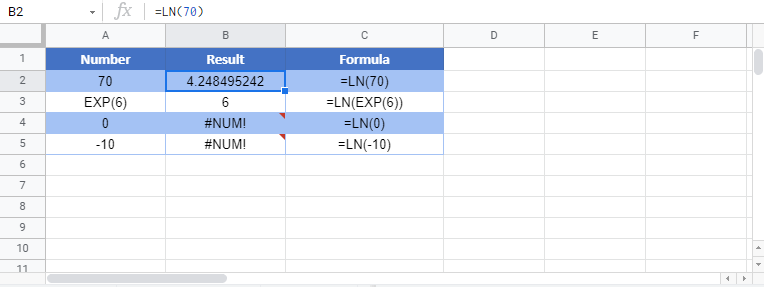Use the LN Function to calculate the natural logarithm of a number. Simply enter any number directly into the formula, or reference a cell containing a number.

## LN Examples in VBA

You can also use the LN function in VBA. Type:
`application.worksheetfunction.ln(number)`
For the function arguments (number, etc.), you can either enter them directly into the function, or define variables to use instead.

will return the natural logarithm of number 5, which is 1.6094

Also, we can use an existing cell as an input parameter, so if we enter the following VBA command

and the value in cell A1 is 4, we will get the following result: